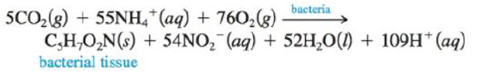# Bacterial digestion is an economical method of sewage treatment. The reaction is an intermediate step in the conversion of the nitrogen in organic compounds into nitrate ions. What mass of bacterial tissue is produced in a treatment plant for every 1.0 × 104 kg of wastewater containing 3.0% NH 4 + ions by mass? Assume that 95% of the ammonium ions are consumed by the bacteria.### Chemistry: An Atoms First Approach

2nd Edition
Steven S. Zumdahl + 1 other
Publisher: Cengage Learning
ISBN: 9781305079243

#### Solutions

Chapter
Section### Chemistry: An Atoms First Approach

2nd Edition
Steven S. Zumdahl + 1 other
Publisher: Cengage Learning
ISBN: 9781305079243
Chapter 5, Problem 111E
Textbook Problem
1369 views

## Bacterial digestion is an economical method of sewage treatment. The reactionis an intermediate step in the conversion of the nitrogen in organic compounds into nitrate ions. What mass of bacterial tissue is produced in a treatment plant for every 1.0 × 104 kg of wastewater containing 3.0% NH4+ ions by mass? Assume that 95% of the ammonium ions are consumed by the bacteria.

Interpretation Introduction

Interpretation: The mass of the bacterial tissue that is produced in the treatment plant for every 1.0×104kg of wastewater containing 3.0%NH4+ ions by mass.

Concept introduction: The mass of a substance can be obtained by using the number of moles of the substance present and its molar mass. The formula used to calculate the mass of a given substance is,

Massofthesubstance=(Numberofmoles)×(Molarmassofthesubstance)

To determine: The mass of the bacterial tissue produced in the treatment plant for every 1.0×104kg of wastewater.

### Explanation of Solution

Given

The stated chemical equation is,

5CO2(g)+55NH4+(aq)+76O2(g)bacteriaC5H7O2N(s)+54NO2(aq)+52H2O(l)+109H+(aq)

The given mass of waste water is 1.0×104kg .

The mass of NH4+ ions is 3% of the mass of waste water.

Therefore, the mass of NH4+ ions =(3100×1.0×104)kg=300kg

It is assumed that 95% of the NH4+ ions react.

Therefore, the mass of the reacted NH4+ ions =(95100×300)kg=285kg=285000g

Molar mass of NH4+ =N+4H=(14+(4×1))g/mol=18g/mol

Formula

The number of moles of a substance is calculated by the formula,

Numberofmoles=GivenmassofthesubstanceAtomicmassofthesubstance

Substitute the value of the given mass and the atomic mass of NH4+ in the above expression.

NumberofmolesofNH4+=285000g18g/mol=15833

### Still sussing out bartleby?

Check out a sample textbook solution.

See a sample solution

#### The Solution to Your Study Problems

Bartleby provides explanations to thousands of textbook problems written by our experts, many with advanced degrees!

Get Started

Find more solutions based on key concepts
The Academy of Nutrition and Dietetics recommends fluoride-free water for the U.S. population. T F

Nutrition: Concepts and Controversies - Standalone book (MindTap Course List)

Speed is a(n) ___ quantity. (2.2)

An Introduction to Physical Science

What is the difference between our Solar System, our galaxy, and the Universe?

Horizons: Exploring the Universe (MindTap Course List)

Oceanography: An Invitation To Marine Science, Loose-leaf Versin

Why is the following situation impossible? Emily challenges David to catch a 1 bill as follows. She holds the b...

Physics for Scientists and Engineers, Technology Update (No access codes included)

Why cant a spacecraft go beyond Earths gravity?

Foundations of Astronomy (MindTap Course List)

Find the electric flux through the three Gaussian surfaces in Figure 25.12.

Physics for Scientists and Engineers: Foundations and Connections